# B: Calculate the distance between two points without using a coordinate system

• Trysse
XYZ and ΔABX still exist, but AB is no longer a vector.In summary, the problem is not a home-work problem. I have come up with this question for myself. Nevertheless, I am stuck and need your help.

#### Trysse

Homework Statement
Imagine you have five points on a flat plane. Name the points A, B, X, Y and Z. The position is arbitrary but fixed. The points X, Y and Z act as reference points. Points A and B are just some other points. Imagine now, that you know the following distances: The distances between the reference points X, Y and Z: XY, XZ, YZ. The distance of each reference point to points A and B: XA, YA, ZA, XB, YB, ZB.

Calculate the distance between points A and B.
Relevant Equations
a^2+b^2=c^2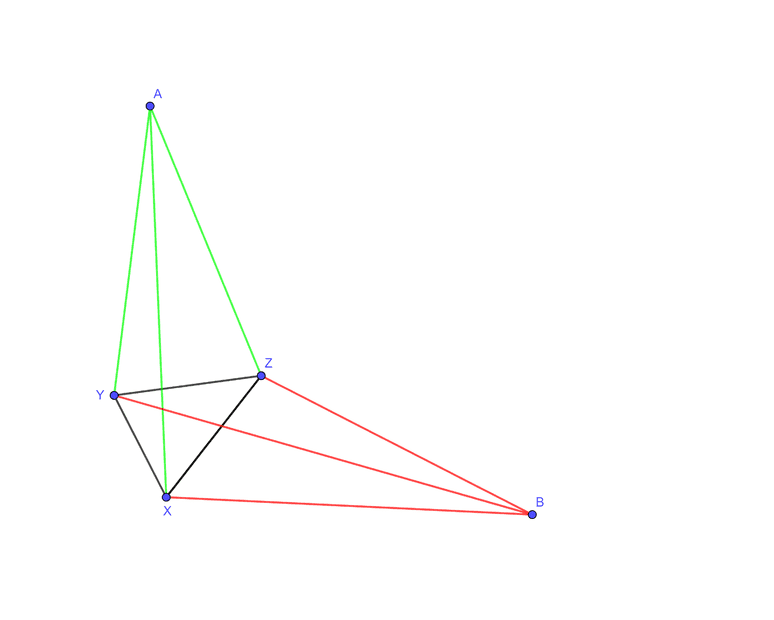Dear all,

the following problem is not a home-work problem. I have come up with this question for myself. Nevertheless, I am stuck and need your help.

The question is: Can I calculate the distance between points A and B from this information? And if yes, how?

I think it should be possible. However, I do not know how to go about it.

These are my considerations so far:

The points X, Y, and Z form a kind of reference frame. Every point P on the plane is described by a unique combination of distances between the point and the three other points.

However, the difference is the following: In a coordinate system with two axes x and y, any combination of values for x and y will always return a valid point. In the case of the reference frame X, Y, and Z only very special combinations of three numbers/distances describe a point. Geometrically this can be shown as three circles around the points X, Y, and Z. Only those combinations of circles, that intersect in one point, define one specific point P. All other combinations do not describe a point. Inversely this can be drawn as three concentric circles around the point P where each of the circles goes through one of the fixed points.

In a cartesian coordinate system, I can also add this extra parameter in the form of the distance between the origin (0,0) (or any other arbitrary and the point P (x,y) that I want to describe. However, this additional distance adds no further information.

In a cartesian coordinate system, I can convert the two-dimensional coordinates (x,y) of a point P into three distances. A simple example would be:

• the distance between the P and the origin (0,0)
• the distance between P and the point (1,0)
• the distance between P and the point (0,1)
I can calculate the distance between P and those points using the Theorem of Pythagoras.

However, in my problem, the distances between the three points X, Y, and Z in the basic problem are not (necessarily) 1 and there are not (necessarily) any right angles.

It is also possible to write all those distances in the form of vectors and matrices, however, this gave me no further idea.

I also had the hunch to I think of the distances as radii of circles. If I do so, I can subtract the areas of those circles and calculate the radii of the resulting circles. However, by trying to do so I got lost and none of the results I got was meaningful. But I still think this is the way to go…

I think this problem cannot be new, but I have no idea where to look for a solution and I am stuck with my own ideas.

#### Attachments

Have tried to reproduce the shape on a paper using only a compass?

Trysse said:
I think this problem cannot be new, but I have no idea where to look for a solution and I am stuck with my own ideas.
Possibly the fact that you have three reference points is adding to the confusion. You can almost get by with only two (for instance, X and Y). The third point just allows you to disambiguate between the two distinct sides of line XY.

With just the length of the base of a triangle (line segment XY) and the lengths of two sides (XA and YA) can you compute the perpendicular distance from line XY to point A?

jbriggs444 said:
Possibly the fact that you have three reference points is adding to the confusion. You can almost get by with only two (for instance, X and Y). The third point just allows you to disambiguate between the two distinct sides of line XY.
The reason I have chosen three points of reference is exactly as you say: With two points of reference X and Y, the position of another point A is not (fully) defined on a plane. For every pair of distances XA and YA, there are two possible positions of A relative to the X and Y. So, with the distances XA, YA, XB, and YB, the situation is not fully described.

Trysse said:
Calculate the distance between points A and B.
Consider, for example, ΔABX. Let θ= ∠AXB.

Since AX and BX are known, we can find AB using the cosine rule providing we can first find cos(θ).

θ is the sum of ∠AXZ and ∠ZXB and the cosine (and thence sine) of each of these can be found from the known lengths and cosine rule.

There may be some special cases to consider, e.g. if A and/or B are inside ΔXYZ.

Hi @Steve4Physics

the hint to the cosine rule was helpful. However, I am not interested in this specific case depicted in the diagram or in any other specific case. I am looking for the most general solution,
If I ignore the distances YA and YB, I cannot know from the other distances only, what the figure looks like, For example, point B could have two positions. See the below diagram at B'.

Maybe I must rephrase my question:

Is it possible to calculate the distance between two points A and B on a plane without any further consideration if the following information is given?
• Distance between A and three arbitrary points X, Y, and Z
• Distance between B and the same three points X,Y, and Z
• Distances between the points X, Y, and Z
In a way, I am looking for an algorithm/equation to calculate the distance AB, the same way I am able to calculate the distance between A and B with the Theorem of Pythagoras in a cartesian coordinate system.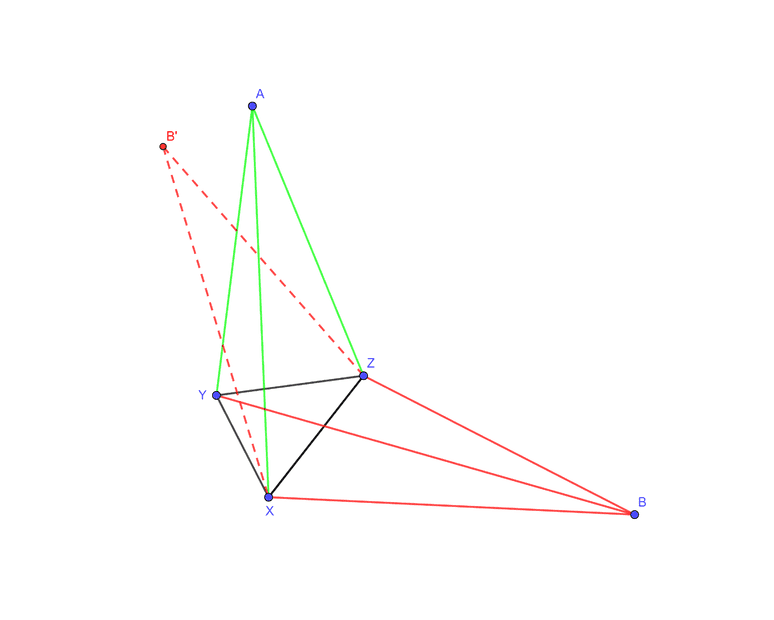Hi @Steve4Physics

the hint to the cosine rule was helpful. However, I am not interested in this specific case depicted in the diagram or in any other specific case. I am looking for the most general solution,
If I ignore the distances YA and YB, I cannot know from the other distances only, what the figure looks like, For example, point B could have two positions. See the below diagram at B'.

Maybe I must rephrase my question:

Is it possible to calculate the distance between two points A and B on a plane without any further consideration if the following information is given?
• Distance between A and three arbitrary points X, Y, and Z
• Distance between B and the same three points X,Y, and Z
• Distances between the points X, Y, and Z
In a way, I am looking for an algorithm/equation to calculate the distance AB, the same way I am able to calculate the distance between A and B with the Theorem of Pythagoras in a cartesian coordinate system.

View attachment 299556

Trysse said:
In a way, I am looking for an algorithm/equation to calculate the distance AB, the same way I am able to calculate the distance between A and B with the Theorem of Pythagoras in a cartesian coordinate system.
A few thoughts…

My suggested (Post #5) approach could be modified. For example, two formulae for AB could be derived - one by considering ΔABX and the other by considering ΔABY.

Then, possibly, from these two formulae, a third formula (or algorithm) could be constructed which satisfies both of the initial two formulae and contains all the given distances. This third formulae (or algorithm) would be the general one you want.

Also, can I note that it is risky to assume that a single formula (analogous to the theorem of Pythagoras) exists for your system. It may – but it’s a big assumption.

I believe you need to consider all potentially distinct cases.

The ‘topology’ (though not quite the right word) changes depending whether or not A and/or B are inside/outside ΔXYZ Assuming the existence of a single formula/algorithm which works in all situations seems optimistic!

•Trysse
Trysse said:
three arbitrary points X, Y, and Z
Not arbitrary. X, Y and Z must not be colinear.

•hutchphd and Trysse
jbriggs444 said:
Not arbitrary. X, Y and Z must not be colinear.
Yes. You are right. XYZ must be a triangle i.e. not colinear.

@Trysse, if you are still working on this, maybe the following will help.

Consider a quadrilateral with vertices labelled 1, 2, 3 and 4. The six distances (four sides and two diagonals) are related, according to https://mathworld.wolfram.com/Quadrilateral.html, by: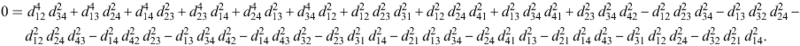(I don’t know if any restrictions apply – e, g. if the quadrilateral must be convex. You would need to do further research.)

In your Post #1 diagram, consider the quadrilateral XYAB. Five of the quadrilateral’s six distances are known; the unknown distance is AB, which we can call ‘L’,

We can now get an equation of the form L⁴ + bL² + c = 0 [Edit: should aL⁴ + bL² + c = 0, I mistyped and omitted the 'a'] which is easily solved. If there is only a single positive solution, presumably that’s the unique value of L and point Z is redundant; if not, the procedure must somehow be extended to include point Z.

#### Attachments

Last edited:
@Steve4Physics
Wow. Yes. On a first glance the equation you have posted looks like what I was thinking about: Taking squares of distances and somehow adding and subtracting them. But I was not expecting to see anything raised beyond the power of two.
Steve4Physics said:
L⁴ + bL² + c = 0
What are b and c in your equation?

The equation you took from Wolfram.com shows, why it is so difficult to think about the relation between six distances. It is just crowded. And I find it difficult to keep an overview.

I will definitely look into this. Thanks a lot.

If ever anything comes out of this I will report it here.

Two things occur belatedly occur to me.

1. This must be a well-studied area since it is, in two dimensions, the same problem faced by GPS receivers in four dimensions. It follows that any advice that I can give from first principles is likely better understood by others already.

2. Since the problem is over-constrained, rather than solving equations, one should be looking for a best-fit position that fits some possibly erroneous inputs. A quick trip to Google says that "yes, that is how it is done".

https://www.serialsjournals.com/abstract/40748_ch_67_-_58._revathi-new.pdf
("Precise Positioning of GPS Receivers using
Least Squares Method")

•Trysse and hutchphd
Trysse said:
What are b and c in your equation?
Please note that in Post #11 I mistyped ‘L⁴ + bL² + c = 0.’ It should have been
aL⁴ + bL² + c = 0.
I’ve updated the Post to correct this.

Suppose L (the unknown length of AB) corresponds to ##d_{12}## in the Wolfram notation.

(Note that Wolfram also sometimes use ##d_{21}## for the same distance, in order to maintain the ‘cyclic patterns’ of the subscripts.)

The other 5 lengths, ##d_{23}, d_{34}, d_{41}, d_{13}## and ##d_{24}##, are known values. When you put these values into the Wolfram equation you will end up with (just making up some numbers randomly) something like this:

##3.2d_{12}^4 + 3.1 + 5.3 + 7.2 + 5.5 + 9.1d_{12}^2 + 8.7d_{12}^2 + 6.3d_{12}^2 + 1.1 + 7.0 - 1.2d_{12}^2##
## - 3.4 - 5.1d_{12}^2 - 8.8 - 7.9 - 6.6 - 4.3 - 8.1d_{12}^2 - 8.2 - 8.9d_{12}^2 -1.0d_{12}^2 - 6.6d_{12}^2 = 0##

When you group the terms together this simplifies to
##ad_{12}^4 + bd_{12}^2 + c = 0## where
##a = 3.2##
##b = 9.1 + 8.7 + 6.3 - 1.2 ...##
##c =3.1 + 5.3 + 7.2 …##
That's where the a, b and c in 'aL⁴ + bL² + c = 0' come from.

•Trysse
jbriggs444 said:
1. This must be a well-studied area since it is, in two dimensions, the same problem faced by GPS receivers in four dimensions. It follows that any advice that I can give from first principles is likely better understood by others already.
I agree. I was actually hoping, that someone would just say: "This is how it is done".
jbriggs444 said:
2. Since the problem is over-constrained, rather than solving equations, one should be looking for a best-fit position that fits some possibly erroneous inputs. A quick trip to Google says that "yes, that is how it is done".
I am not sure about the statement, that "the problem is over-constrained".

At a first glance, it might appear over-constrained to use three distances to describe the location of a point on a plane. However, this is because we are used to defining the location of a point in a coordinate system with only two parameters. But what is missing in that consideration is, that the coordinate system is also a constraint in itself:
• Distances must only be expressed in certain directions
• The directions must be at certain angles to each other.
• Some directions must be expressed with negative numbers
For some reason, these constraints (or maybe rather conventions) free us from the need to know a third distance. This might be the reason, why we do not see them as constraints.

From a practical point of view, the two-axis-coordinate system is probably way easier to use than the three-point-coordinate system.

The reason why I found the idea of the three-point-coordinates interesting in the first place was the fact, that a three-point-coordinate does not require the concept of direction. Taken from a plane into space, this means: With four reference points in space, I can describe all other points in space.

Anyway, at the moment I come to the conclusion that it might be more difficult to calculate the distance than I originally thought it might be.

Trysse said:
I am not sure about the statement, that "the problem is over-constrained".
Consider point A alone. You have three measured distances to point A. Two measurements are adequate to reduce point A's location to one of two possibilities. The third measured distance must have one of two possible values, exactly.

If your measurements are subject to error, they may not be compatible with one another. They may "over-constrain" the system so that no solution exists that exactly fits all of the constraints.

The problem is then one of deciding the most probable location of point A, given the possibly inexact measurements that were supplied.

Waving my hands wildly and with no real expertise in the area, one might try to minimize the sum of squared measurement errors required to get an exact fit.

Check out my implementation at https://www.desmos.com/calculator/9qpqekeqvx
[ slight modification: https://www.desmos.com/calculator/rgeqfdc3ob ]
(move the points around!)

[See further update (described below the horizontal line): https://www.desmos.com/calculator/rdwho5iodf ]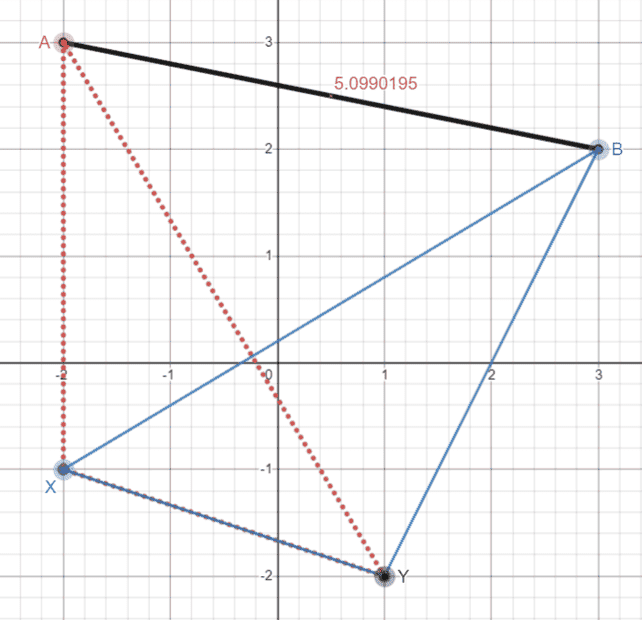I implemented the formula posted by @Steve4Physics (derived from the Cayley-Merger Determinant) from https://mathworld.wolfram.com/Quadrilateral.html
(See the formula below in MathJax. Note that Desmos uses LaTeX-like notation, when copy-pasting.
Since I already typed it in, it's easier for you to do something else with it.)

The 4 points A,B,X,Y yield the 6 lines that are featured in the determinant.
I evaluated that determinant, which gives ##0\pm10^{-10}##.

Then I did a regression in Desmos for a variable ##Q## which plays the role of the "distance from A to B" if "0=determinant" with that ##Q## (treated as free) is solved for.

Compare that ##Q## (from regression) with Desmos's distance function.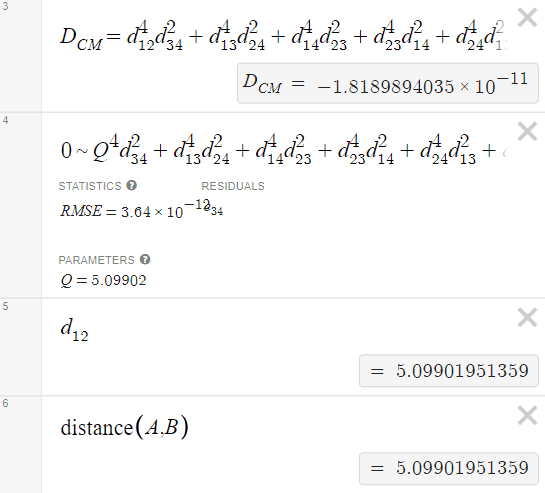(pasted from Desmos... reformatted manually)
NOTE: These expression can be simplified because the distance functions are symmetric: ##d_{21}=d_{12}##.
They were likely written without using this fact in order to systematically generate all of the appropriate terms... keep track of them.

So, for doing algebra and solving,
one can essentially reduce the number of terms by about half.

An orderly method is to: write any term ##d_{ba}## with ##b>a## as "##d_{ab}##".
##
\begin{align*}
D_{CM}
&=&
d_{12}^{4}d_{34}^{2}+d_{13}^{4}d_{24}^{2}+d_{14}^{4}d_{23}^{2}+d_{23}^{4}d_{14}^{2}+d_{24}^{4}d_{13}^{2}+d_{34}^{4}d_{12}^{2}\\
&&
+d_{12}^{2}d_{23}^{2}d_{31}^{2}+d_{12}^{2}d_{24}^{2}d_{41}^{2}+d_{13}^{2}d_{34}^{2}d_{41}^{2}+d_{23}^{2}d_{34}^{2}d_{42}^{2}
\\
&&
-d_{12}^{2}d_{23}^{2}d_{34}^{2}-d_{13}^{2}d_{32}^{2}d_{24}^{2}-d_{12}^{2}d_{24}^{2}d_{43}^{2}-d_{14}^{2}d_{42}^{2}d_{23}^{2}-d_{13}^{2}d_{34}^{2}d_{42}^{2}-d_{14}^{2}d_{43}^{2}d_{32}^{2}\\
&&-d_{23}^{2}d_{31}^{2}d_{14}^{2}-d_{21}^{2}d_{13}^{2}d_{34}^{2}-d_{24}^{2}d_{41}^{2}d_{13}^{2}-d_{21}^{2}d_{14}^{2}d_{43}^{2}-d_{31}^{2}d_{12}^{2}d_{24}^{2}-d_{32}^{2}d_{21}^{2}d_{14}^{2}
\end{align*}
##

## 0\sim Q^{4}d_{34}^{2}+d_{13}^{4}d_{24}^{2}+d_{14}^{4}d_{23}^{2}+d_{23}^{4}d_{14}^{2}+d_{24}^{4}d_{13}^{2}+d_{34}^{4}Q^{2}+Q^{2}d_{23}^{2}d_{31}^{2}+Q^{2}d_{24}^{2}d_{41}^{2}+d_{13}^{2}d_{34}^{2}d_{41}^{2}+d_{23}^{2}d_{34}^{2}d_{42}^{2}-Q^{2}d_{23}^{2}d_{34}^{2}-d_{13}^{2}d_{32}^{2}d_{24}^{2}-Q^{2}d_{24}^{2}d_{43}^{2}-d_{14}^{2}d_{42}^{2}d_{23}^{2}-d_{13}^{2}d_{34}^{2}d_{42}^{2}-d_{14}^{2}d_{43}^{2}d_{32}^{2}-d_{23}^{2}d_{31}^{2}d_{14}^{2}-Q^{2}d_{13}^{2}d_{34}^{2}-d_{24}^{2}d_{41}^{2}d_{13}^{2}-Q^{2}d_{14}^{2}d_{43}^{2}-d_{31}^{2}Q^{2}d_{24}^{2}-d_{32}^{2}Q^{2}d_{14}^{2}##

It turns out that it's better to express this in terms of squared-distances.
As in
##
\begin{align*}
D_{CMS}
&=&S_{12}^{2}S_{34}+S_{13}^{2}S_{24}+S_{14}^{2}S_{23}+S_{23}^{2}S_{14}+S_{24}^{2}S_{13}+S_{34}^{2}S_{12}\\
&&+S_{12}S_{23}S_{31}+S_{12}S_{24}S_{41}+S_{13}S_{34}S_{41}+S_{23}S_{34}S_{42}\\
&&-S_{12}S_{23}S_{34}-S_{13}S_{32}S_{24}-S_{12}S_{24}S_{43}-S_{14}S_{42}S_{23}-S_{13}S_{34}S_{42}-S_{14}S_{43}S_{32}\\
&&-S_{23}S_{31}S_{14}-S_{21}S_{13}S_{34}-S_{24}S_{41}S_{13}-S_{21}S_{14}S_{43}-S_{31}S_{12}S_{24}-S_{32}S_{21}S_{14}
\end{align*}
##

So, that Desmos can do its regression (Q is now the squared-distance)
##
0\sim Q^{2}S_{34}+S_{13}^{2}S_{24}+S_{14}^{2}S_{23}+S_{23}^{2}S_{14}+S_{24}^{2}S_{13}+S_{34}^{2}Q+QS_{23}S_{31}+QS_{24}S_{41}+S_{13}S_{34}S_{41}+S_{23}S_{34}S_{42}-QS_{23}S_{34}-S_{13}S_{32}S_{24}-QS_{24}S_{43}-S_{14}S_{42}S_{23}-S_{13}S_{34}S_{42}-S_{14}S_{43}S_{32}-S_{23}S_{31}S_{14}-QS_{13}S_{34}-S_{24}S_{41}S_{13}-QS_{14}S_{43}-S_{31}QS_{24}-S_{32}QS_{14}
##

I gave this problem to Maple and asked for an algebraic solution.
After a lot of text-processing (which I did manually, but probably should write a script for),

I got one root as
(which is implemented in the second update of the original Desmos file)
##
\begin{align*}
Q_{alg}
&=&
\frac{1}{2}(\frac{1}{S_{34}})(
S_{13} S_{34}+S_{14} S_{32}+S_{14} S_{43}-S_{23} S_{31}
+S_{23} S_{34}+S_{24} S_{31}
\\
&&
-S_{24} S_{41}+S_{24} S_{43}
-S_{34}^{2}
\\
&&
-
(-4 S_{13}^{2} S_{24} S_{34}
+S_{13}^{2} S_{34}^{2}
+2 S_{13} S_{14} S_{32} S_{34}
\\
&&
+2 S_{13} S_{14} S_{34} S_{43}
-2 S_{13} S_{23} S_{31} S_{34}
+2 S_{13} S_{23} S_{34}^{2}
\\
&&

-4 S_{13} S_{24}^{2} S_{34}
+2 S_{13} S_{24} S_{31} S_{34}
+4 S_{13} S_{24} S_{32} S_{34}
\\
&&
+2 S_{13} S_{24} S_{34} S_{41}
+2 S_{13} S_{24} S_{34} S_{43}
-2 S_{13} S_{34}^{3}
\\
&&

-4 S_{13} S_{34}^{2} S_{41}
+4 S_{13} S_{34}^{2} S_{42}
-4 S_{14}^{2} S_{23} S_{34}
\\
&&

+S_{14}^{2} S_{32}^{2}
+2 S_{14}^{2} S_{32} S_{43}
+S_{14}^{2} S_{43}^{2}
\\
&&
-4 S_{14} S_{23}^{2} S_{34}
-2 S_{14} S_{23} S_{31} S_{32}
+4 S_{14} S_{23} S_{31} S_{34}
\\
&&

-2 S_{14} S_{23} S_{31} S_{43}
+2 S_{14} S_{23} S_{32} S_{34}
+4 S_{14} S_{23} S_{34} S_{42}
\\
&&
+2 S_{14} S_{23} S_{34} S_{43}
+2 S_{14} S_{24} S_{31} S_{32}
+2 S_{14} S_{24} S_{31} S_{43}
\\
&&
-2 S_{14} S_{24} S_{32} S_{41}
+2 S_{14} S_{24} S_{32} S_{43}
-2 S_{14} S_{24} S_{41} S_{43}
\\
&&
+2 S_{14} S_{24} S_{43}^{2}
-2 S_{14} S_{32} S_{34}^{2}
+4 S_{14} S_{32} S_{34} S_{43}
\\
&&
-2 S_{14} S_{34}^{2} S_{43}
+S_{23}^{2} S_{31}^{2}
-2 S_{23}^{2} S_{31} S_{34}
\\
&&
+S_{23}^{2} S_{34}^{2}
-2 S_{23} S_{24} S_{31}^{2}
+2 S_{23} S_{24} S_{31} S_{34}
\\
&&
+2 S_{23} S_{24} S_{31} S_{41}
-2 S_{23} S_{24} S_{31} S_{43}
-2 S_{23} S_{24} S_{34} S_{41}
+2 S_{23} S_{24} S_{34} S_{43}
\\

&&
+2 S_{23} S_{31} S_{34}^{2}
-2 S_{23} S_{34}^{3}
-4 S_{23} S_{34}^{2} S_{42}
+S_{24}^{2} S_{31}^{2}
-2 S_{24}^{2} S_{31} S_{41}
\\

&&
+2 S_{24}^{2} S_{31} S_{43}
+S_{24}^{2} S_{41}^{2}
-2 S_{24}^{2} S_{41} S_{43}
+S_{24}^{2} S_{43}^{2}
\\

&&
-2 S_{24} S_{31} S_{34}^{2}
+2 S_{24} S_{34}^{2} S_{41}
-2 S_{24} S_{34}^{2} S_{43}+S_{34}^{4}

)^{\frac{1}{2}}
\\
&&
)
\end{align*}
##
(Hopefully I didn't mangle the re-formatting.)
You can get the original from the second-updated Desmos page.

Code:
(1/2)*S34*(S13*S34+S14*S32+S14*S43-S23*S31+S23*S34+S24*S31-S24*S41+S24*S43-S34^2
-(-4*S13^2*S24*S34+S13^2*S34^2+2*S13*S14*S32*S34+2*S13*S14*S34*S43-2*S13*S23*S31*S34+2*S13*S23*S34^2-4*S13*S24^2*S34+2*S13*S24*S31*S34+4*S13*S24*S32*S34+2*S13*S24*S34*S41+2*S13*S24*S34*S43-2*S13*S34^3-4*S13*S34^2*S41+4*S13*S34^2*S42-4*S14^2*S23*S34+S14^2*S32^2+2*S14^2*S32*S43+S14^2*S43^2-4*S14*S23^2*S34-2*S14*S23*S31*S32+4*S14*S23*S31*S34-2*S14*S23*S31*S43+2*S14*S23*S32*S34+4*S14*S23*S34*S42+2*S14*S23*S34*S43+2*S14*S24*S31*S32+2*S14*S24*S31*S43-2*S14*S24*S32*S41+2*S14*S24*S32*S43-2*S14*S24*S41*S43+2*S14*S24*S43^2-2*S14*S32*S34^2+4*S14*S32*S34*S43-2*S14*S34^2*S43+S23^2*S31^2-2*S23^2*S31*S34+S23^2*S34^2-2*S23*S24*S31^2+2*S23*S24*S31*S34+2*S23*S24*S31*S41-2*S23*S24*S31*S43-2*S23*S24*S34*S41+2*S23*S24*S34*S43+2*S23*S31*S34^2-2*S23*S34^3-4*S23*S34^2*S42+S24^2*S31^2-2*S24^2*S31*S41+2*S24^2*S31*S43+S24^2*S41^2-2*S24^2*S41*S43+S24^2*S43^2-2*S24*S31*S34^2+2*S24*S34^2*S41-2*S24*S34^2*S43+S34^4)^(1/2)
)

Last edited:
I will be honest: (1) I didn’t follow closely your original post, (2) I read a handful of comments and start scrolling (on my phone’s touchscreen) like a little kid playing with TeePee in a dispenser.

I have two suggestions for your further personal study: (1) try doing some geometric constructions the only use compass and straightedge—to this end download the free geometric drawing software GeoGebra on your phone or laptop. (2) read up on Stereographic Projection I think you’ll find it fascinating (though typically one does not study this concept until either multidimensional calculus or complex analysis).

Are you in school? HS or college?

jbriggs444 said:
2. Since the problem is over-constrained, rather than solving equations, one should be looking for a best-fit position that fits some possibly erroneous inputs. A quick trip to Google says that "yes, that is how it is done".

https://www.serialsjournals.com/abstract/40748_ch_67_-_58._revathi-new.pdf
("Precise Positioning of GPS Receivers using
Least Squares Method")
Note that this is an evolving area that is much more complicated than that article indicates: see for instance this publicly available paper.

@robphy I am impressed and I must confess I am lost. I have locked at the Desmos-implementation without understanding/knowing how Desmos works. How does the formula "recognize, if the two points A and B are on the same side of the line going through the points X and Y or if they are different sides?

@jbriggs444 yes, the accuracy of measurement with three distances on a plane is an issue I have also seen. And even if I assume, that I can make measurements without error, I have the problem with irrational numbers. How can I measure $sqrt{2}$ or any other irrational number? I had the idea, that I can measure by sending signals back and forth between points. I can count, how often a signal goes back and forth between for example between point $P_1$ and $P_2$ while another signal goes back and forth between $P_1$ and $P_3$. I than get a ration between these tow numbers. The longer I measure, the more accurate my measurement gets
Maybe it is necessary to define an accuracy for calculations. Which will lead to further errors.

@benorin I did the original drawing with GeoGebra. I a kind of a mature student at university (so college, but we don't call it that way here).

pbuk said:
Note that this is an evolving area that is much more complicated than that article indicates:
@pbuk The"evolving" and "much more complicated" made me feel slightly better. Thank you.

However, what I have gotten out of all your answers is, that calculating the distance between the two points involves far more computation than I was expecting. And the whole thing is not as straight forward as I was expecting.

I think I will take a break here, as this is taking up more of my intellectual capacity than I should spent on this topic. I think I will sit down and post here, what made me think of this problem in the first place. This might shed some light, on how I came across this question. But not today. And it might be a bit lengthy... So if you care, stand by. Otherwise: Thank you all for taking the time and putting in effort!

All I did was use the webpage and formulas that @Steve4Physics provided.
In Desmos, I created 4 points (controlled by 8 sliders... x and y for each point).
I defined a functions that correspond to the Pythagorean-theorem-distances between specific points (to be used in the formulas provided by Steve4Physics).

One claim from the webpage is that a certain function of all of those distances add up to zero.
(It's the determinant of a particular matrix where particular functions of these distances appear.
The determinant measures the volume of a tetrahedron, given its lengths. But since all four points lie on a plane, the tetrahedron is flat and its volume is zero.) So, we are checking it with our knowledge of all the Pythagorean-distances.

From this idea, one can leave out one variable (the distance from A to B) and solve for it
in terms of all of the other distances (taken to be "known", via the Pythagorean-distance).
That's what the "regression" does... solve numerically for the distance from A to B using that formula.

Along the lines of what Steve4Physics suggested trying to solve a quadratic formula,
I used Maple (which can do algebra symbolically),
and asked it to write (the distance from A to B) as an explicit formula
in terms of all of the distances (using the formulas provided by Steve4Physics).

So, I entered that as a formula for Desmos to evaluate.

So, I got Desmos to
• verify the zero value of the determinant (the volume of the flattened tetrahedron) up to tiny numerical errors using all distances as known from Pythaogras.
• solve numerically for the unknown (distance from A to B) in terms of all other distances-in-terms-of-Pythagoras.
• evaluate an algebraic formula (gotten by Maple) to get the (distance from A to B) in terms of all other distances-in-terms-of-Pythagoras.
• allow you to check that the numbers agree as you move the points around the plane.

So, in response to your
Imagine now, that you know the following distances: The distances between the reference points X, Y and Z: XY, XZ, YZ. The distance of each reference point to points A and B: XA, YA, ZA, XB, YB, ZB.

Calculate the distance between points A and B.
I took the formula provided by [B]Steve4Physics[/B]
and did just as you asked (but out the need for a third point Z).

Once could use "X and Z" or "Y and Z" instead of "X and Y".

If there are experimental errors or doubts about the measurements,
one could use a third point Z or more points
and then use some kind of averaging (as suggested by jbriggs444).

@robphy I am impressed and I must confess I am lost. I have locked at the Desmos-implementation without understanding/knowing how Desmos works. How does the formula "recognize, if the two points A and B are on the same side of the line going through the points X and Y or if they are different sides?

Note that this formula calculates a distance (not a displacement, with components or with magintude-and-direction).

One thing to note.. the algebraic formula gives two roots.
It appears that when AB crosses XY, the featured root disagrees with the expected answer. Instead the other root agrees. So, some more analysis is probably needed.

Last edited: